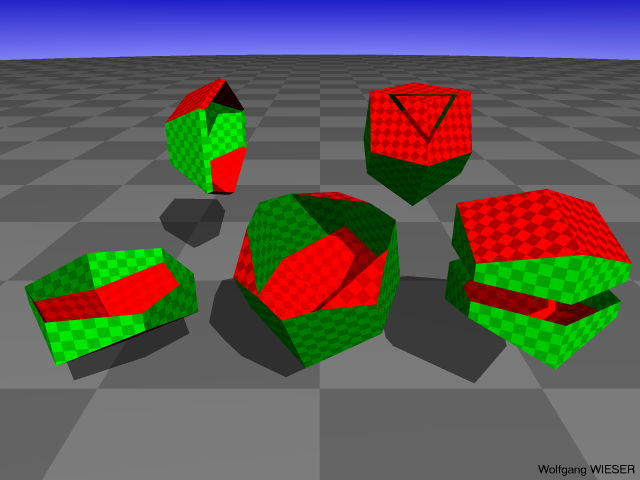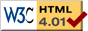Nav: [home] > [render] > [images] > [maths]

## Rendered images of Mathematical Functions

### Schrödinger's Equation

Two solutions for Schrödinger's equation for the hydrogen atom. These are the n=3, l=1, m=0 and n=3, l=2, m=0 electron location probability isosurfaces with a0 set to 1 at a threshold of 0.00008.
These images were rendered by me using POVRay.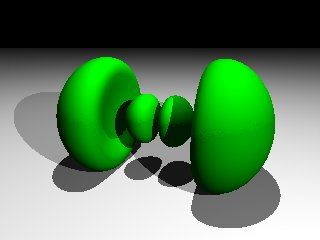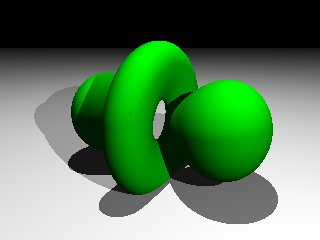This was the latest color and clipping experiment:### Wave Guide

The next images do not show solutions of Schrödinger's equation :) They show the strength of the magnetic field in the transversal cut of a cylindrical wave guide; more precisely it visualizes the center part of the transverse magnetic mode m=2 (first image) and m=7 (second image).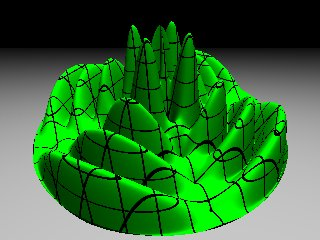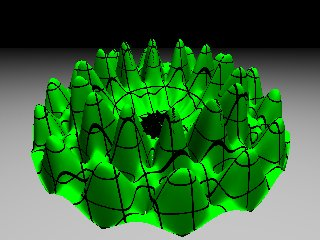These images were created using a self-patched version of POVRay with added support for the Bessel functions.
Here is a larger image for m=3.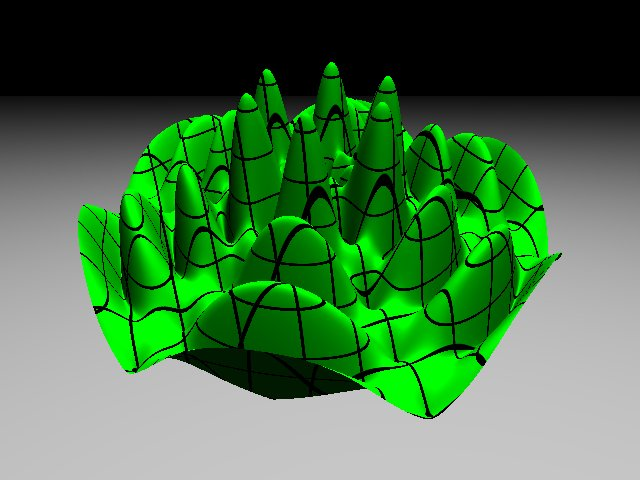Note that this shows only the center part of the wave, i.e. the border of the green wave in the image is not the inner border of the cylindrical wave guide. (Oh, I just did not take care to adjust the radius properly 'cause it was too late at night.)

### Wigner-Seitz Cell of HCP structure